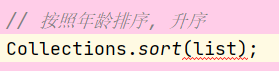# 常用功能

java.util.Collections是集合工具类, 用来对集合进行操作. 部分方法如下:

• public static <T> boolean addAll(Collection<T> c, T... elements): 往集合中添加一些元素
• public static void shuffle(List<?> list): 打乱顺集合顺序
• public static <T> void sort(List<T> list): 将集合中元素按照默认规则排序
• public static <T> void sort(List<T> list，Comparator<? super T> ): 将集合中元素按照指定规则排序

import java.util.ArrayList;
import java.util.Collections;

public class Test43 {
public static void main(String[] args) {
ArrayList<Integer> list = new ArrayList<>();
// 原来的写法

// 采用工具类完成往集合中添加元素
System.out.println(list);

// 排序方法
Collections.sort(list);
System.out.println(list);
}
}

[6, 1, 2, 3, 4, 5]
[1, 2, 3, 4, 5, 6]


# Comparator 比较器

public static <T> void sort(List<T> list，Comparator<? super T> )


import java.util.ArrayList;
import java.util.Collections;

public class Test44 {
public static void main(String[] args) {
ArrayList<String> arrayList = new ArrayList<>();

// 排序方法
Collections.sort(arrayList);
System.out.println(arrayList);
}
}

[6, 1, 2, 3, 4, 5]
[1, 2, 3, 4, 5, 6]


public final class String implements java.io.Serializable, Comparable<String>, CharSequence {


String 类实现了这个接口, 并完成了比较规则的定义, 但是这样就把这种规则写死了. 那比如我想要字符串按照第一个字符降序排列就要修改 String 源代码, 这是不可能的. 那么这个时候我们就可以使用public static <T> void sort(List<T> list，Comparator<? super T> )方法灵活完成. 这个里面就涉及到了 Comparator 这个接口, 位于java.util包下. 排序是 comparator 能实现的功能之一, 该接口代表一个比较器, 比较器具有可比性! 顾明思议就是做排序的, 通俗地讲需要比较两个对象谁排在前面谁排在后面. 那么比较方法就是:

public int compare(String o1, String o2) ：


import java.util.ArrayList;
import java.util.Collections;
import java.util.Comparator;

public class Test {
public static void main(String[] args) {
ArrayList<String> list = new ArrayList<>();

// 排序方法, 按照第一个单词的降序
Collections.sort(list, new Comparator<String>() {
@Override
public int compare(String o1, String o2) {
return o2.charAt(0) - o1.charAt(0);
}
});
System.out.println(list);
}
}

[sba, nba, cba, aba]


# 简述 Compareable 和 Comparator 两个接口的区别

## Comparable

Compara: 强行对实现它的每个类的对象进行整体排序. 这种排序被称为类的自然排序, 类的 compareTo 方法被称为它的自然比较方法. 只能在类中实现 compareTo() 一次, 不能经常修改类的代码实现自己想要的排序. 实现此接口的对象列表 (和数组) 可以通过 Collections.sort (和 Arrays.sort) 进行自动排序. 对象可以作有序映射中的键或有序集合中的元素, 无需指定比较器.

## Comparator

Comparator: 强行对某个对象进行整体排序. 可以将 Comparator 传递给 sort 方法 ( 如 Collections.sort 或 Arrays.sort ), 从而允许在排序上实现精准控制. 还可以使用 Comparator 来控制某些数据结构 ( 如有序 set 或有序映射 ) 的顺序, 或者为那些没有自然顺序的对象 collection提供排序.

# 练习

## Student 初始类

public class Student {
private String name;
private int age;

public Student(){

}

public Student(String name, int age){
this.name = name;
this.age = age;
}

public String getName() {
return name;
}

public void setName(String name) {
this.name = name;
}

public int getAge() {
return age;
}

public void setAge(int age) {
this.age = age;
}

@Override
public String toString() {
return "Student{" +
"name='" + name + '\'' +
", age=" + age +
'}';
}
}


## 测试类

import java.util.ArrayList;
import java.util.Collections;
import java.util.Comparator;

public class Test2 {
public static void main(String[] args) {
// 创建四个学生对象
ArrayList<Student> list = new ArrayList<>();

// 按照年龄排序, 升序
Collections.sort(list);

System.out.println(list.toString());
}
}public class Student implements Comparable<Student>{
...
@Override
public int compareTo(Student o) {
// 升序
return this.age - o.age;
}
}


Student{name='武藤兰', age=18}
Student{name='小泽玛利亚', age=19}
Student{name='苍井空', age=20}
Student{name='马保国', age=69}


# 扩展

Collections.sort(list, new Comparator<Student>() {
@Override
public int compare(Student o1, Student o2) {
return o2.getAge() - o1.getAge();  // 以学生的年龄降序
}
});


Collections.sort(list, new Comparator<Student>() {
@Override
public int compare(Student o1, Student o2) {
// 年龄降序
int result = o2.getAge() - o1.getAge();//年龄降序
if(result == 0){  // 第一个规则判断完了 下一个规则, 姓名的首字母升序
result = o1.getName().charAt(0) - o2.getName().charAt(0);
}
return result;
}
});